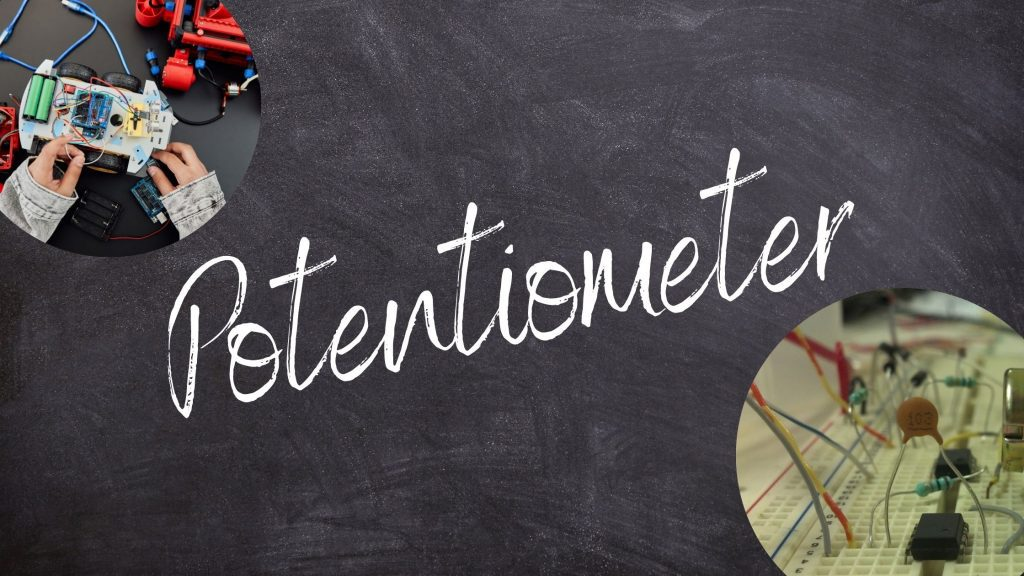Physics
Study Material

# Potentiometer

Potentiometer measures potential difference more accurate as compared to voltmeter. This instrument works on null point method.

2 minutes long
Posted by Mahak Jain, 27/10/2021Hesap Oluştur

Got stuck on homework? Get your step-by-step solutions from real tutors in minutes! 24/7. Unlimited.

Potentiometer measures potential difference more accurate as compared to voltmeter but voltmeter is used more often due to its small size and convenience.

## Basic Structure of Potentiometer

• Wire AB is few meters long and have uniform cross section area.
• Potential gradient = V/L = K……..(1)         [ as the x increase the potential difference across x also increases but the ratio of v/x is constant = V/L = K ]
• The practical look of potentiometer is quite different, which is given below.

## Uses of Potentiometer

Potentiometer has manily three uses, which are given below.

To measure EMF of cell : Here, first we will find a null point on wire AB where galvanometer(G) shows no deflections, as shown in below figure.

Let C be the point on wire AB where G shows no deflection  which means there is no current in branch ADYC.

It means that, the potential difference accross cell and potential difference across AC is same.

So,  potential drop across AC = (V/L)x = Kx

So, Kx = E(cell EMF)

Thus, EMF of cell is,  E = (V/L)x Or E = Kx

To compare EMF of two cell : By this we can measure EMF of one cell if EMF of other cell is given.

If circuit DE is closed and FP is open then we get null point for E1, as shown in below figure.

so,         E1 = (V/L)(x1) ……….. (1)

• And if circuit DE is open and FG is closed then we get null point at for E2

so,        E2 = (V/L)(x2)………..(2)

• By dividing equation (1) and (2) we get,

E1/E2 = x1/x2

So,            E = (V/L)(x1)
(V/L)(x1) = E ……..(1)

Now look at the circuit CDFQ given below, here current will flow due to cell. So potenital drop in CD is

-E+ir = 0
Hence,           E-ir = 0 …………(2)

If QF and CD both are closed then null point is obtained at length x2.

So,          (V/L)(x2) = E-ir
So,          (V/L)(x2) = E-[Er/(R+r)] = E[(R+r-r)/(R+r)]
Thus,          (V/L)(x2) = ER/(R+r) ………..(3)

By dividing (1) and (2) we get,

(x1/x2) = (R+r)/R
Hence            r = R[(x1/x2) -1]

Note :

Polarity of Battery should not be opposite to cell.

Potential drop accross the potentiometer wire (Wire AB) should be greater then EMF of cell.